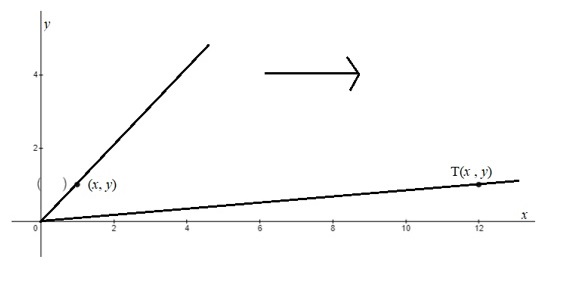# (a) identify the transformation, and (b) graphically represent the transformkhi1la2f1qv 2021-11-21 Answered
(a) identify the transformation, and
(b) graphically represent the transformation for an arbitrary vector
in ${R}^{2}$.
T(x, y) = (12x, y)
You can still ask an expert for help

• Live experts 24/7
• Questions are typically answered in as fast as 30 minutes
• Personalized clear answers

Solve your problem for the price of one coffee

• Math expert for every subject
• Pay only if we can solve itSue Leahy
Step 1
(a) It is given that T(x,y)=(12x.y).
Step 2
For a given transformation T(x,y) =(kx,y), the horizontal expansion occurs when k > 1.
Here k=12(>1)
Thus, the given linear transformation is a horizontal expansion.

We have step-by-step solutions for your answer!Sact1989

Step 3
(b) Here
$T\left(11\right)=\left(12\cdot 1,1\right)$
=(12,1)
Graph the given linear transformation as shown below:We have step-by-step solutions for your answer!